Related Articles

# Ukkonen’s Suffix Tree Construction – Part 1

• Difficulty Level : Hard
• Last Updated : 13 Feb, 2018

Suffix Tree is very useful in numerous string processing and computational biology problems. Many books and e-resources talk about it theoretically and in few places, code implementation is discussed. But still, I felt something is missing and it’s not easy to implement code to construct suffix tree and it’s usage in many applications. This is an attempt to bridge the gap between theory and complete working code implementation. Here we will discuss Ukkonen’s Suffix Tree Construction Algorithm. We will discuss it in step by step detailed way and in multiple parts from theory to implementation. We will start with brute force way and try to understand different concepts, tricks involved in Ukkonen’s algorithm and in the last part, code implementation will be discussed.
Note: You may find some portion of the algorithm difficult to understand while 1st or 2nd reading and it’s perfectly fine. With few more attempts and thought, you should be able to understand such portions.

Book Algorithms on Strings, Trees and Sequences: Computer Science and Computational Biology by Dan Gusfield explains the concepts very well.

A suffix tree T for a m-character string S is a rooted directed tree with exactly m leaves numbered 1 to m. (Given that last string character is unique in string)

• Root can have zero, one or more children.
• Each internal node, other than the root, has at least two children.
• Each edge is labelled with a nonempty substring of S.
• No two edges coming out of same node can have edge-labels beginning with the same character.

Concatenation of the edge-labels on the path from the root to leaf i gives the suffix of S that starts at position i, i.e. S[i…m].

Note: Position starts with 1 (it’s not zero indexed, but later, while code implementation, we will used zero indexed position)

For string S = xabxac with m = 6, suffix tree will look like following: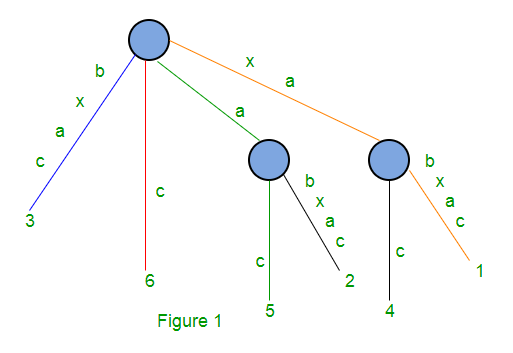It has one root node and two internal nodes and 6 leaf nodes.

String Depth of red path is 1 and it represents suffix c starting at position 6
String Depth of blue path is 4 and it represents suffix bxca starting at position 3
String Depth of green path is 2 and it represents suffix ac starting at position 5
String Depth of orange path is 6 and it represents suffix xabxac starting at position 1

Edges with labels a (green) and xa (orange) are non-leaf edge (which ends at an internal node). All other edges are leaf edge (ends at a leaf)

If one suffix of S matches a prefix of another suffix of S (when last character in not unique in string), then path for the first suffix would not end at a leaf.

For String S = xabxa, with m = 5, following is the suffix tree: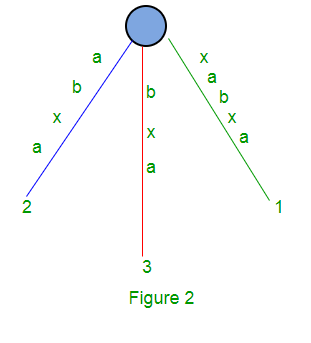Here we will have 5 suffixes: xabxa, abxa, bxa, xa and a.
Path for suffixes ‘xa’ and ‘a’ do not end at a leaf. A tree like above (Figure 2) is called implicit suffix tree as some suffixes (‘xa’ and ‘a’) are not seen explicitly in tree.

To avoid this problem, we add a character which is not present in string already. We normally use \$, # etc as termination characters.
Following is the suffix tree for string S = xabxa\$ with m = 6 and now all 6 suffixes end at leaf.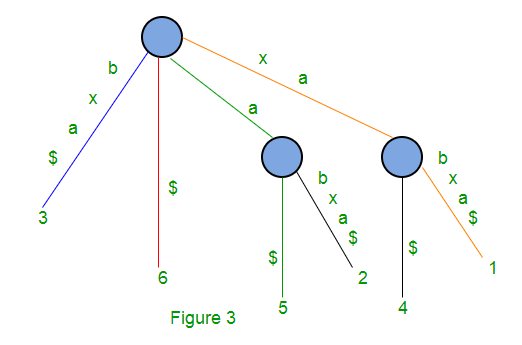A naive algorithm to build a suffix tree
Given a string S of length m, enter a single edge for suffix S[l ..m]\$ (the entire string) into the tree, then successively enter suffix S[i..m]\$ into the growing tree, for i increasing from 2 to m. Let Ni denote the intermediate tree that encodes all the suffixes from 1 to i.
So Ni+1 is constructed from Ni as follows:

• Start at the root of Ni
• Find the longest path from the root which matches a prefix of S[i+1..m]\$
• Match ends either at the node (say w) or in the middle of an edge [say (u, v)].
• If it is in the middle of an edge (u, v), break the edge (u, v) into two edges by inserting a new node w just after the last character on the edge that matched a character in S[i+l..m] and just before the first character on the edge that mismatched. The new edge (u, w) is labelled with the part of the (u, v) label that matched with S[i+1..m], and the new edge (w, v) is labelled with the remaining part of the (u, v) label.
• Create a new edge (w, i+1) from w to a new leaf labelled i+1 and it labels the new edge with the unmatched part of suffix S[i+1..m]

This takes O(m2) to build the suffix tree for the string S of length m.
Following are few steps to build suffix tree based for string “xabxa\$” based on above algorithm: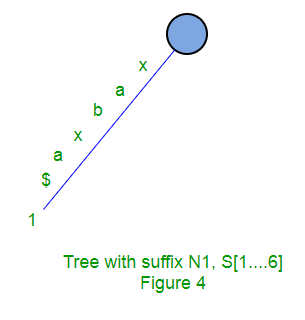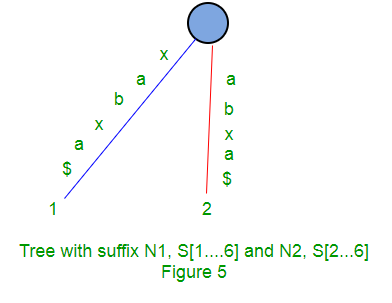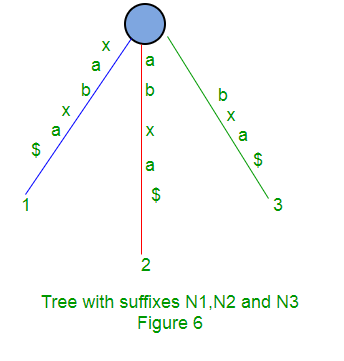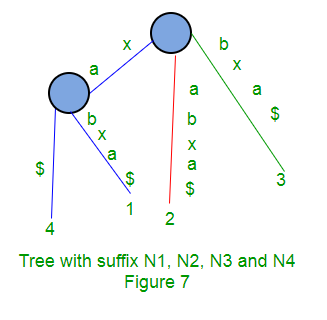Implicit suffix tree
While generating suffix tree using Ukkonen’s algorithm, we will see implicit suffix tree in intermediate steps few times depending on characters in string S. In implicit suffix trees, there will be no edge with \$ (or # or any other termination character) label and no internal node with only one edge going out of it.
To get implicit suffix tree from a suffix tree S\$,

• Remove all terminal symbol \$ from the edge labels of the tree,
• Remove any edge that has no label
• Remove any node that has only one edge going out of it and merge the edges.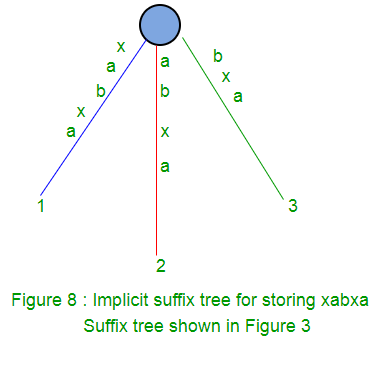High Level Description of Ukkonen’s algorithm
Ukkonen’s algorithm constructs an implicit suffix tree Ti for each prefix S[l ..i] of S (of length m).
It first builds T1 using 1st character, then T2 using 2nd character, then T3 using 3rd character, …, Tm using mth character.
Implicit suffix tree Ti+1 is built on top of implicit suffix tree Ti.
The true suffix tree for S is built from Tm by adding \$.
At any time, Ukkonen’s algorithm builds the suffix tree for the characters seen so far and so it has on-line property that may be useful in some situations.
Time taken is O(m).

Ukkonen’s algorithm is divided into m phases (one phase for each character in the string with length m)
In phase i+1, tree Ti+1 is built from tree Ti.

Each phase i+1 is further divided into i+1 extensions, one for each of the i+1 suffixes of S[1..i+1]
In extension j of phase i+1, the algorithm first finds the end of the path from the root labelled with substring S[j..i].
It then extends the substring by adding the character S(i+1) to its end (if it is not there already).
In extension 1 of phase i+1, we put string S[1..i+1] in the tree. Here S[1..i] will already be present in tree due to previous phase i. We just need to add S[i+1]th character in tree (if not there already).
In extension 2 of phase i+1, we put string S[2..i+1] in the tree. Here S[2..i] will already be present in tree due to previous phase i. We just need to add S[i+1]th character in tree (if not there already)
In extension 3 of phase i+1, we put string S[3..i+1] in the tree. Here S[3..i] will already be present in tree due to previous phase i. We just need to add S[i+1]th character in tree (if not there already)
.
.
In extension i+1 of phase i+1, we put string S[i+1..i+1] in the tree. This is just one character which may not be in tree (if character is seen first time so far). If so, we just add a new leaf edge with label S[i+1].

High Level Ukkonen’s algorithm
Construct tree T1
For i from 1 to m-1 do
begin {phase i+1}
For j from 1 to i+1
begin {extension j}
Find the end of the path from the root labelled S[j..i] in the current tree.
Extend that path by adding character S[i+l] if it is not there already
end;
end;

Suffix extension is all about adding the next character into the suffix tree built so far.
In extension j of phase i+1, algorithm finds the end of S[j..i] (which is already in the tree due to previous phase i) and then it extends S[j..i] to be sure the suffix S[j..i+1] is in the tree.

There are 3 extension rules:
Rule 1: If the path from the root labelled S[j..i] ends at leaf edge (i.e. S[i] is last character on leaf edge) then character S[i+1] is just added to the end of the label on that leaf edge.

Rule 2: If the path from the root labelled S[j..i] ends at non-leaf edge (i.e. there are more characters after S[i] on path) and next character is not s[i+1], then a new leaf edge with label s{i+1] and number j is created starting from character S[i+1].
A new internal node will also be created if s[1..i] ends inside (in-between) a non-leaf edge.

Rule 3: If the path from the root labelled S[j..i] ends at non-leaf edge (i.e. there are more characters after S[i] on path) and next character is s[i+1] (already in tree), do nothing.

One important point to note here is that from a given node (root or internal), there will be one and only one edge starting from one character. There will not be more than one edges going out of any node, starting with same character.

Following is a step by step suffix tree construction of string xabxac using Ukkonen’s algorithm: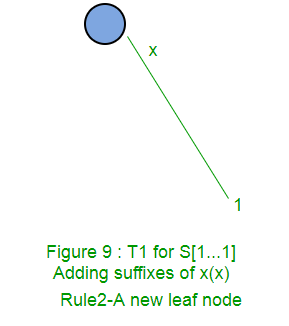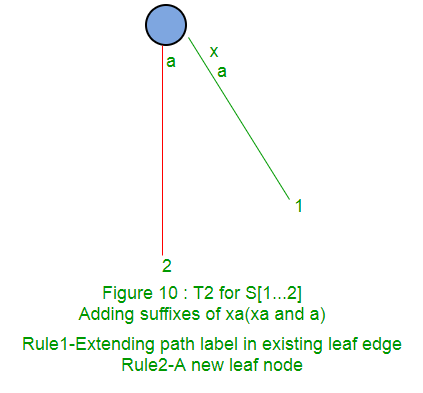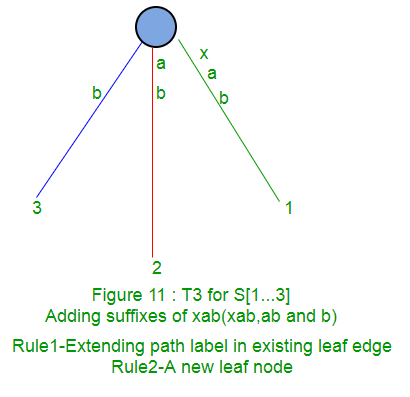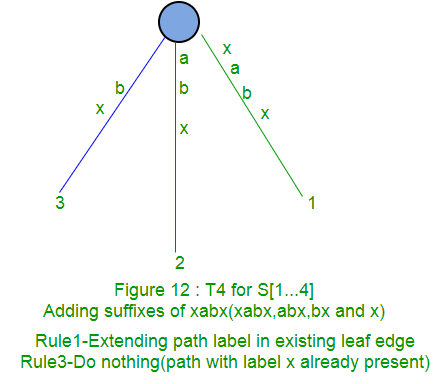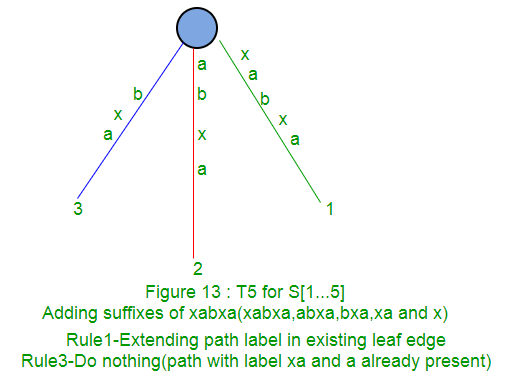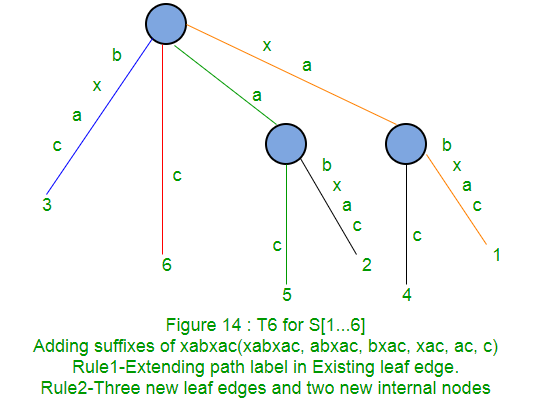In next parts (Part 2, Part 3, Part 4 and Part 5), we will discuss suffix links, active points, few tricks and finally code implementations (Part 6).

References:
http://web.stanford.edu/~mjkay/gusfield.pdf